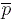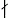Ramanujan-type Congruences for Overpartitions Modulo 16

William Y.C. Chen, Qing-Hu Hou, Lisa H. Sun and Li Zhang

 Abstract:  Let(n) denote the number of overpartitions of n. Recently, Fortin-Jacob-Mathieu and Hirschhorn-Sellers independently obtained 2-, 3- and 4-dissections of the generating function for(n) and derived a number of congruences for(n) modulo 4, 8 and 64 including(8n+7) ≡ 0 (mod 64), for n ≥ 0. In this paper, we give a 16-dissection of the generating function for(n) modulo 16 and show that(16n+14) ≡ 0 (mod 16) for n ≥ 0. Moreover, using the 2-adic expansion of the generating function for(n) according to Mahlburg, we obtain that(l2n + rl) ≡ 0 (mod 16), where n ≥ 0, l ≡ -1 (mod 8) is an odd prime and r is a positive integer with lr. In particular, for l = 7 and n ≥ 0, we get(49n+7) ≡ 0 (mod 16) and(49n+14) ≡ 0 (mod 16). We also find four congruence relations:(4n) ≡ (-1)n(n) (mod 16) for n ≥ 0,(4n) ≡ (-1)n(n) (mod 32) where n is not a square of an odd positive integer,(4n) ≡ (-1)n(n) (mod 64) for n1, 2, 5 (mod 8) and(4n) ≡ (-1)n(n) (mod 128) for n ≡ 0 (mod 4).   AMS Classification:  05A17, 11P83   Keywords:  overpartition, Ramanujan-type congruence, 2-adic expansion, dissection formula   Download:   pdf

Return